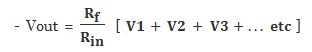Summing Amplifier

Theory

Op-amp Summing Amplifier CircuitFig.1 Summing Amplifier Circuit

An adder circuit can be made by connecting more inputs to the inverting Op-amp. The opposite end of the resistor connected to the inverting input is held at virtual ground by the feedback; therefore, adding new inputs does not affect the response of the existing inputs.

The Summing Amplifier is a very flexible circuit based upon the standard Inverting Operational Amplifier configuration that can be used for combining multiple inputs. The inverting amplifier has a single input voltage, ( Vin ) applied to the inverting input terminal, If we add more input resistors to the input, each equal in value to the original input resistor, Rin we end up with another operational amplifier circuit called a Summing Amplifier, "summing inverter" or even a "voltage adder".

We now have an operational amplifier circuit that will amplify each individual input voltage and produce an output voltage signal that is proportional to the algebraic "SUM" of the three individual input voltages V1, V2 and V3. We can also add more inputs if required as each individual input "see's" their respective resistance, Rin as the only input impedance. This is because the input signals are effectively isolated from each other by the "virtual earth" node at the inverting input of the Op-amp. A direct voltage addition can also be obtained when all the resistances are of equal value and Rƒ is equal to Rin.